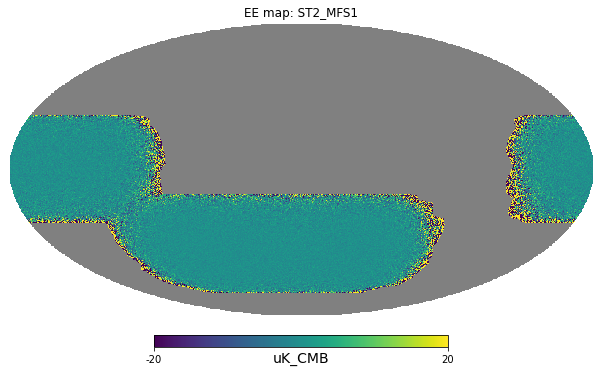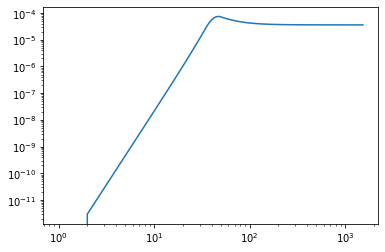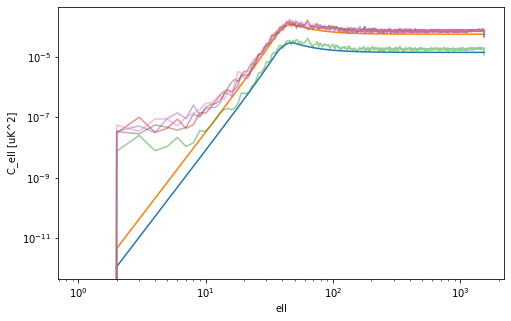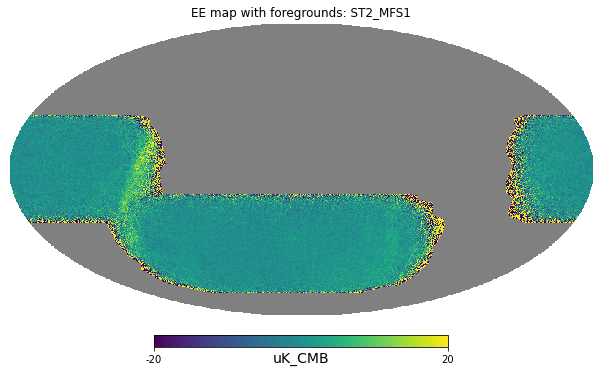In :
import mapsims
import numpy as np
import healpy as hp
import pysm3.units as u
from pathlib import Path
%matplotlib inline
import matplotlib.pyplot as plt


# Access existing noise map based simulations¶

The last noise simulation, just released is 202006_noise.

What is different in noise releases is that just 1 realization of full mission and splits is saved on disk, the others can be generated on-the-fly using mapsims, as we will see in the next notebook.

In :
channels = mapsims.parse_channels("tube:ST2")

In :
channel = channels

In :
print(channel)

Channel ST2_MFS1


This release has variable $N_{side}$ based on the channel, see this table, we have a utility function to retrieve that:

In :
nside = mapsims.get_default_so_resolution(channel)


# Load maps from a map based release at NERSC¶

In :
folder = Path("/project/projectdirs/sobs/v4_sims/mbs/")
release = "202006_noise"

In :
filename_template = "{content}/{num:04d}/simonsobs_{content}_uKCMB_{tube}_{band}_nside{nside}_{num:04d}_{split}_of_{nsplits}.fits"

In :
filenames = [Path(folder) / release / filename_template.format(nside=nside, content="noise", num=0,
telescope=channel.telescope, tube=channel.tube, band=channel.band,
split=1, nsplits=1)]

In :
for split in range(1, 5):
filenames += [Path(folder) / release / filename_template.format(nside=nside, content="noise", num=0,
telescope=channel.telescope, tube=channel.tube, band=channel.band,
split=split, nsplits=4)]

In :
maps = [hp.read_map(filename, (0,1,2)) for filename in filenames]

/global/common/software/sobs/mbs/lib/python3.7/site-packages/healpy/fitsfunc.py:352: UserWarning: If you are not specifying the input dtype and using the default np.float64 dtype of read_map(), please consider that it will change in a future version to None as to keep the same dtype of the input file: please explicitly set the dtype if it is important to you.
"If you are not specifying the input dtype and using the default "

NSIDE = 512
ORDERING = RING in fits file
INDXSCHM = IMPLICIT
NSIDE = 512
ORDERING = RING in fits file
INDXSCHM = IMPLICIT
NSIDE = 512
ORDERING = RING in fits file
INDXSCHM = IMPLICIT
NSIDE = 512
ORDERING = RING in fits file
INDXSCHM = IMPLICIT
NSIDE = 512
ORDERING = RING in fits file
INDXSCHM = IMPLICIT

In :
hp.mollview(maps, min=-20, max=20, title="EE map: " + channel.tag, unit="uK_CMB")# Load the theoretical noise curve¶

Using mapsims, we can load the noise spectra generated from the so_noise_models package. By default they are generated for the full sky, therefore we need to scale down by the sky fraction to get the expected noise for a partial sky survey.

In :
noise = mapsims.SONoiseSimulator(nside=nside)

In :
ell, ps_T, ps_P = noise.get_fullsky_noise_spectra(tube=channel.tube)

In :
plt.loglog(ell, ps_P);# Load hitmaps, apodize and take spectra¶

Noise is very inhomogeneous, a direct anafast would be dominated by the border pixels with just a few hits, the trick is to multiply by the hitmap and then renormalize by the mean of the hitmap squared (as the $C_\ell$ are in power).

The sky fraction instead is needed because anafast always takes a transform of the full sky setting 0 on all the missing pixels. Therefore the spectrum power is averaged down and we need to scale back by the sky fraction.

In :
hitmaps, ave_nhits = noise.get_hitmaps(tube=channel.tube)

/global/common/software/sobs/mbs/lib/python3.7/site-packages/mapsims/utils.py:71: UserWarning: Access data from /global/cfs/cdirs/sobs/www/so_mapsims_data/v0.2/healpix/ST2_MFS1_01_of_20.nominal_telescope_all_time_all_hmap.fits.gz
warnings.warn(f"Access data from {full_path}")
/global/common/software/sobs/mbs/lib/python3.7/site-packages/mapsims/utils.py:71: UserWarning: Access data from /global/cfs/cdirs/sobs/www/so_mapsims_data/v0.2/healpix/ST2_MFS2_01_of_20.nominal_telescope_all_time_all_hmap.fits.gz
warnings.warn(f"Access data from {full_path}")
/global/common/software/sobs/mbs/lib/python3.7/site-packages/healpy/fitsfunc.py:352: UserWarning: If you are not specifying the input dtype and using the default np.float64 dtype of read_map(), please consider that it will change in a future version to None as to keep the same dtype of the input file: please explicitly set the dtype if it is important to you.
"If you are not specifying the input dtype and using the default "

In :
sky_fraction = (hitmaps > 0).sum() / len(hitmaps)

In :
sky_fraction

Out:
0.3847748438517253
In :
# using iter=0 to speed it up, for production runs set use_pixel_weights=True
cls = [hp.anafast(m * hitmaps, iter=0) / np.mean(hitmaps**2) / sky_fraction for m in maps]

In :
plt.figure(figsize=(8, 5))
plt.loglog(ell, ps_P * sky_fraction)
plt.loglog(ell, ps_P * sky_fraction * 4)

for cl in cls:
plt.loglog(cl, alpha=.5)
plt.xlabel("ell")
plt.ylabel("C_ell [uK^2]");Currently the noise simulations are at variable $N_{side}$ while the foregrounds and CMB are 512/4096, so we might need to use hp.ud_grade. We are lucky that this channel is already at 512 so we do not need to.

The next foreground release will also be at variable $N_{side}$ so this issue will not apply anymore.

In :
folder = "/project/projectdirs/sobs/v4_sims/mbs/201906_highres_foregrounds_extragalactic_tophat"

In :
filename_template = "{nside}/{content}/{num:04d}/simonsobs_{content}_uKCMB_{telescope}{band}_nside{nside}_{num:04d}.fits"

In :
filename = Path(folder) / filename_template.format(nside=512, content="combined", num=0,
telescope=channel.telescope.lower(), band=channel.band)

In :
foregrounds_cmb = hp.ma(hp.read_map(filename, (0,1,2)))

/global/common/software/sobs/mbs/lib/python3.7/site-packages/healpy/fitsfunc.py:352: UserWarning: If you are not specifying the input dtype and using the default np.float64 dtype of read_map(), please consider that it will change in a future version to None as to keep the same dtype of the input file: please explicitly set the dtype if it is important to you.
"If you are not specifying the input dtype and using the default "

NSIDE = 512
ORDERING = NESTED in fits file
INDXSCHM = IMPLICIT
Ordering converted to RING
Ordering converted to RING
Ordering converted to RING

In :
maps = [each + foregrounds_cmb for each in maps]

In :
hp.mollview(maps, min=-20, max=20, title="EE map with foregrounds: " + channel.tag, unit="uK_CMB")In [ ]: